# Inverse Proportion

Here we will learn about inverse proportion, including what inverse proportion is and how to solve inverse proportion problems including real-life problem solving. We will also have a look at the inverse proportionality formula.

There are also inverse proportion worksheets based on Edexcel, AQA and OCR exam questions, along with further guidance on where to go next if you’re still stuck.

## What is inverse proportion?

Inverse proportion is a type of proportionality relationship. If two quantities are inversely proportional then as one quantity increases, the other decreases.

An example of inverse proportion would be the hours of work required to build a wall. If there are more people building the same wall, the time taken to build the wall reduces.

Conversely, an example of direct proportion would be that the area of a circle is directly proportional to its radius.

Inverse proportion is also known as indirect proportion or inverse variation.

Inverse proportion is applied to real life problems such as the speed of a moving object, determining whether an item will float or sink in water, or the time taken to complete a finite task whereas direct proportion is useful in numerous real life situations such as exchange rates, conversion between units, and fuel prices.

To determine the value of a variable that is inversely proportional to another, we need to determine the relationship between the two variables and then use this to find our unknown value.

Similar to a directly proportional relationship, we need to determine the constant of proportionality, k.

Step-by-step guide: Direct proportion

### What is inverse proportion?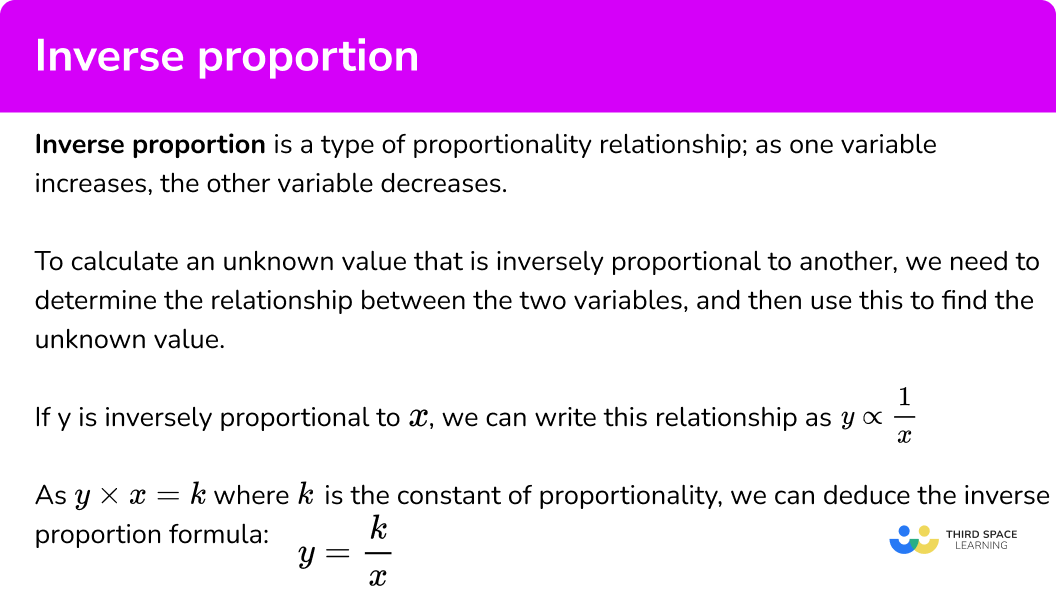### Inverse proportion formula

The symbol \propto is the proportionality symbol and it represents a proportional relationship between two variables. If it is inversely proportional to x, we write this relationship as y\propto\frac{1}{x}.

This relationship can be described using an equivalence relationship. When y is inversely proportional to x , the value of x \times y is a constant value. This value is the constant of proportionality and we use the letter k to denote this value. Using a formula, we have k=xy.

Rearranging this formula to make y the subject, we obtain the inverse proportion formula,

y=\frac{k}{x} .

Step-by-step guide: Inverse proportion formula

### Graphs representing an inverse proportion between two variables

Proportional relationships can also be represented by graphs. If we sketched a graph of the line y=\frac{k}{x}, as x increases in size, k is being divided by a larger number and so the result is a value y that gets increasingly smaller. This gives us the curved line graph of the reciprocal function.

Note, the value of y can be inversely proportional to other powers of x including x^{2}, \ x^{3}, or even \sqrt{x}. Each of these has a different algebraic and graphical representation.

Step-by-step guide: Directly proportional graphs / inversely proportional graphs

## How to use inverse proportion

In order to work out an unknown value given an inversely proportional relationship:

1. Write down the inverse proportion formula.
2. Determine the value of \textbf{k} .
3. Substitute \textbf{k} and the known value into the inverse proportion formula.
4. Solve the equation.

### Explain how to use inverse proportion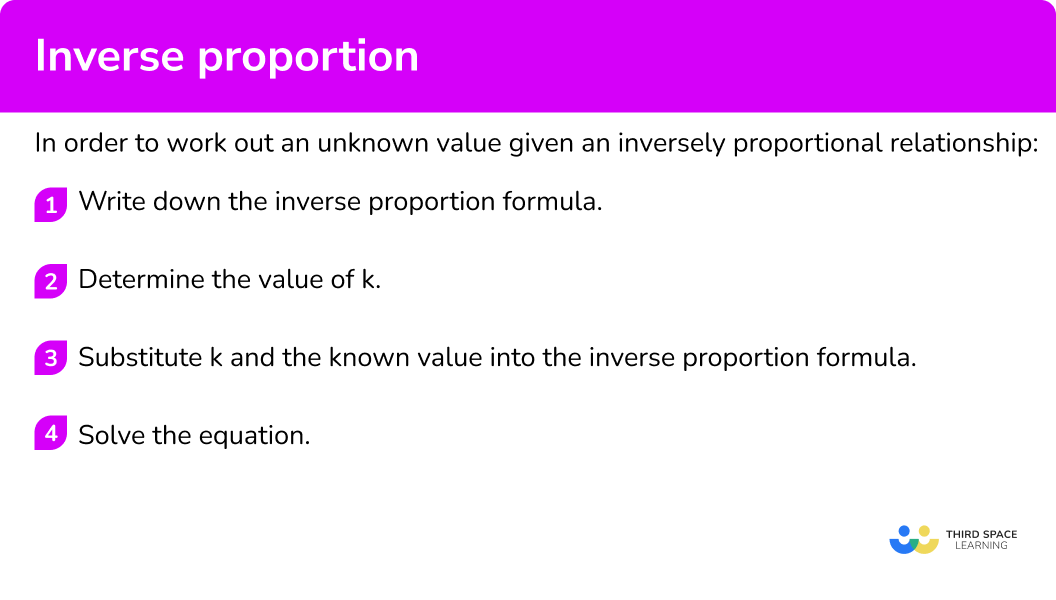## Inverse proportion examples

### Example 1: complete the table y ∝ ⅟x

Given that y is inversely proportional to x, calculate the missing value of y in the table below.

1. Write down the inverse proportion formula.

As y\propto\frac{1}{x}, we can write the formula y=\frac{k}{x}.

2Determine the value of \textbf{k} .

Substituting a known pair of values (3,20), we can say,

\begin{aligned} 20&=\frac{k}{3}\\\\ 20\times{3}&=k\\\\ k&=60 \end{aligned}

3Substitute \textbf{k} and the known value into the inverse proportion formula.

Now we have the equation y=\frac{60}{x}. Substituting x=12 into the equation to calculate the value for y, we have

y=\frac{60}{12} .

4Solve the equation.

Dividing 60 by 12, we have y=5.

Notice that as the value for x increased, the value for y decreased.

### Example 2: complete the table y ∝ ⅟x

Let y\propto\frac{1}{x}. Calculate the value for x when y=20.

Write down the inverse proportion formula.

Determine the value of \textbf{k} .

Substitute \textbf{k} and the known value into the inverse proportion formula.

Solve the equation.

### Example 3: complete the table y ∝ ⅟x2

Let y be inversely proportional to x^{2}. Calculate the missing value q.

Write down the inverse proportion formula.

Determine the value of \textbf{k} .

Substitute \textbf{k} and the known value into the inverse proportion formula.

Solve the equation.

### Example 4: inverse proportion worded problem y ∝ ⅟x

2 workers paint a fence in 9 hours.

How long would it take 6 workers to paint the same fence?

Write down the inverse proportion formula.

Determine the value of \textbf{k} .

Substitute \textbf{k} and the known value into the inverse proportion formula.

Solve the equation.

### Example 5: inverse proportion worded problem y ∝ ⅟x2

Let n represent the number of dogs and d represent the number of days. A bag of biscuits feeds 4 dogs for 12 days. Given that d\propto\frac{1}{n^{2}}, how many days would the same bag feed 5 dogs?

Write down the inverse proportion formula.

Determine the value of \textbf{k} .

Substitute \textbf{k} and the known value into the inverse proportion formula.

Solve the equation.

### Example 6: inverse proportion worded problem y ∝ ⅟x3

A cube of cheese has a density d=1.075\text{g/cm}^{3}. The side length of the cube is x=1.5cm. Another cube of cheese of the same mass has a density d=1.072\text{g/cm}^{3}.

What is the side length of the second cube of cheese? Write your answer to 3 decimal places.

Write down the inverse proportion formula.

Determine the value of \textbf{k} .

Substitute \textbf{k} and the known value into the inverse proportion formula.

Solve the equation.

### Common misconceptions

• Modelling assumption

Whenever you solve word problems for inverse proportion you assume that everything has the same rate. For example, if the question involves the number of people working, we assume all the workers work at the same rate.

• The constant of proportionality

For direct proportion, the constant of proportionality k is the ratio of the two variables such as k=y\div{x}. For inverse proportion, k is the product of the two variables, such as k=xy.

• The graph of an inversely proportional relationship does not cross either axis

The graph of any inversely proportional relationship cannot cross either axis. This is because, if we use the example y\propto\frac{1}{x}, if x=0, the value for y is undefined as we cannot divide a number by 0.

If y=0, then the value for the constant of proportionality must be 0 or x=0, but as we have just said, x 0 and so the relationship between x and y cannot exist for these two values as k=0.

• Take care with writing time using the correct decimal

Time is used in some inverse proportion word problems. If an answer is 3.1 you may be tempted to write it as 3 hours 10 minutes, but it would be 3 hours 6 minutes. (Remember there are 60 minutes in an hour).

• Graphs of the reciprocal function

The standard graph for y\propto{x} is a straight line graph that passes through the origin with the gradient k. The standard graph for y\propto\frac{1}{x} is a curved line that does not cross either axis.

## Related lessons on inverse proportion

Inverse proportion is part of our series of lessons to support revision on proportion. You may find it helpful to start with the main proportion lesson for a summary of what to expect, or use the step by step guides below for further detail on individual topics. Other lessons in this series include:

### Practice inverse proportion questions

1. As y is inversely proportional to x, complete the table by calculating the missing value for y.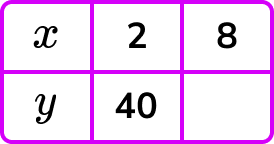160402010y=\frac{k}{x} and so k=xy.

k=2\times{40}=80

y=\frac{80}{x}

When x=8, \ y=\frac{80}{8}=10 .

2. Given that n is inversely proportional to the square of m, calculate the positive value for m when n=8.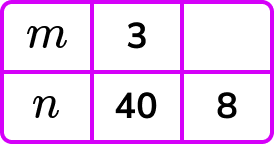-296.7 \ (1d.p.)1513.3 \ (1d.p.)n=\frac{k}{m^2} and so k=m^{2} n.

k=9\times{40}=360

n=\frac{360}{m^2}

When n=8, \ m^2=\frac{360}{8}=45

m=\sqrt{45} = 6.7 (1d.p.)

3. Let b be inversely proportional to \sqrt{a}. Calculate the value for b when a=25.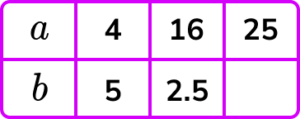1.253.9 \ (1dp)202b=\frac{k}{\sqrt{a}} and so k=b\sqrt{a}.

k=5\times{\sqrt{4}}=5\times{2}=10

b=\frac{10}{\sqrt{a}}

When a=25, \ b=\frac{10}{\sqrt{25}}=\frac{10}{5}=2 .

4. 20 people take 36 days to build a house. How many days would it take 60 people to build the same house?

20 days24 days12 days108 daysLet p represent the number of people and d represent the number of days of building, then

d=\frac{k}{p} and so k=dp.

k=36\times{20}=720

d=\frac{720}{p}

When p=60, \ d=\frac{720}{60}=12.

5. A bike travelling at 8 \ km/h completes a journey in 40 minutes. How long would the same journey take if the speed was increased to 16 \ km/h?

60 minutes20 minutes80 minutes10 minutesLet s represent speed and t represent time taken, then

s=\frac{k}{t} and so k=st.

k=8\times{40}=320

s=\frac{320}{t}

When s=16,

\begin{aligned} 16&=\frac{320}{t}\\\\ 16\times{t}&=320\\\\ t&=320\div{16}\\\\ t&=20 \end{aligned}

Note, the speed-distance-time formula is a known relationship (speed = distance \div time). For a constant distance, if the time taken to reach the destination increases, the speed must have decreased, or vice versa.

6. A fudge company works out that the cost of making a bag of fudge (c) decreases as the number of bags produced (b) increases. If c\propto\frac{1}{b^{2}} and producing 12 bags of fudge costs £2.70 each to make, how many bags of fudge can be made for £1.50 each?

16780259We have c=\frac{k}{b^{2}} and so k=b^{2}c.

k=12^{2}\times{2.7}=388.8

c=\frac{388.8}{b^{2}}

When c=1.5,

\begin{aligned} 1.5&=\frac{388.8}{b^{2}}\\\\ 1.5\times{b^{2}}&=388.8\\\\ b^{2}&=388.8\div{1.5}\\\\ b^{2}&=259.2\\\\ b&=\sqrt{259.2}\\\\ b&=16.09968944…\\\\ b&=16\text{ bags} \end{aligned}

### Inverse proportion GCSE questions

1. Let y be inversely proportional to the square of x.

Complete the table.(4 marks)

k=x^{2}y=36 \times 2=72

(1)

y=\frac{72}{x^{2}}   or  y=72\div{2^{2}}   or  y=72\div{12^{2}}

(1)

18

(1)

0.5

(1)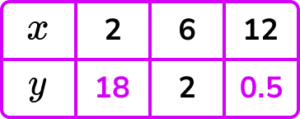2. It takes 6 workers 24 hours to build an emergency flood barrier.

The wall needs to be built in 8 hours.

How many workers are needed to build the same emergency flood barrier in 8 hours?

(2 marks)

6\times 3

(1)

18 workers

(1)

3. It takes 360 minutes to fill a swimming pool from 5 taps.

(a) How many minutes would it take 3 taps to fill the swimming pool?

(3 marks)

(a)

k=xy=360 \times 5=1800

(1)

1800 \div 3=600 minutes

(1)

(b)

All taps work at the same rate.

(1)

## Learning checklist

You have now learned how to:

• Solve problems involving inverse proportion, including algebraic representations

## Still stuck?

Prepare your KS4 students for maths GCSEs success with Third Space Learning. Weekly online one to one GCSE maths revision lessons delivered by expert maths tutors.

Find out more about our GCSE maths tuition programme.